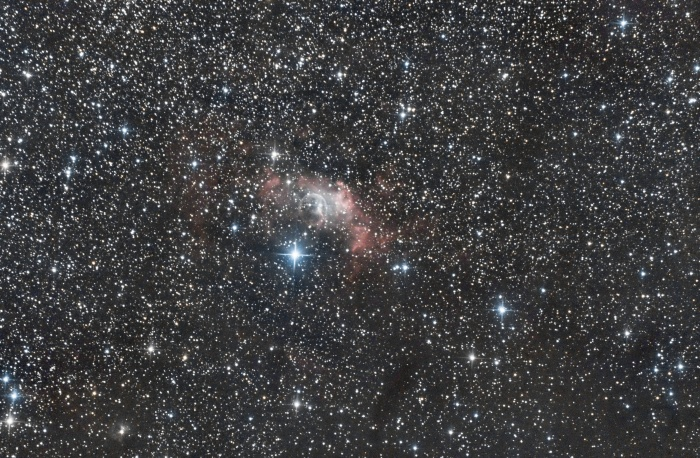Outdated translations are marked like this.
Other languages:
English • ‎español • ‎français

# Siril processing tutorial

## Stacking

The final step to do with Siril is to stack the images. Go to the "stacking" tab, indicate if you want to stack all images, only selected images or the best images regarding the value of FWHM previously computed. Siril proposes several algorithms for stacking computation.

• Sum Stacking

This is the simplest algorithm: each pixel in the stack is summed using 32-bit precision, and the result is normalized to 16-bit. The increase in signal-to-noise ratio (SNR) is proportional to $\sqrt{N}$, where $N$ is the number of images. Because of the lack of normalisation, this method should only be used for planetary processing.

• Average Stacking With Rejection
• Percentile Clipping: this is a one step rejection algorithm ideal for small sets of data (up to 6 images).
• Sigma Clipping: this is an iterative algorithm which will reject pixels whose distance from median will be farthest than two given values in sigma units ($\sigma_{low}$, $\sigma_{high}$).
• Median Sigma Clipping: this is the same algorithm except than the rejected pixels are replaced by the median value of the stack.
• Winsorized Sigma Clipping: this is very similar to Sigma Clipping method but it uses an algorithm based on Huber's work  .
• Linear Fit Clipping: this is an algorithm developed by Juan Conejero, main developer of PixInsight . It fits the best straight line ($y=ax+b$) of the pixel stack and rejects outliers. This algorithm performs very well with large stacks and images containing sky gradients with differing spatial distributions and orientations.

These algorithms are very efficient to remove satellite/plane tracks.

• Median Stacking

This method is mostly used for dark/flat/offset stacking. The median value of the pixels in the stack is computed for each pixel. As this method should only be used for dark/flat/offset stacking, it does not take into account shifts computed during registration. The increase in SNR is proportional to $0.8\sqrt{N}$.

• Pixel Maximum Stacking

This algorithm is mainly used to construct long exposure star-trails images. Pixels of the image are replaced by pixels at the same coordinates if intensity is greater.

• Pixel Minimum Stacking

This algorithm is mainly used for cropping sequence by removing black borders. Pixels of the image are replaced by pixels at the same coordinates if intensity is lower.

In the case of NGC7635 sequence, we first used the "Winsorized Sigma Clipping" algorithm in "Average stacking with rejection" section, in order to remove satellite tracks ($\sigma_{low}=4$ and $\sigma_{high}=3$).

The output console thus gives the following result:

   14:33:06: Pixel rejection in channel #0: 0.181% - 1.184%
14:33:06: Pixel rejection in channel #1: 0.151% - 1.176%
14:33:06: Pixel rejection in channel #2: 0.111% - 1.118%
14:33:06: Integration of 12 images:
14:33:06: Pixel combination ......... average
14:33:06: Normalization ............. additive + scaling
14:33:06: Pixel rejection ........... Winsorized sigma clipping
14:33:06: Rejection parameters ...... low=4.000 high=3.000
14:33:07: Saving FITS: file NGC7635.fit, 3 layer(s), 4290x2856 pixels
14:33:07: Execution time: 9.98 s.
14:33:07: Background noise value (channel: #0): 9.538 (1.455e-04)
14:33:07: Background noise value (channel: #1): 5.839 (8.909e-05)
14:33:07: Background noise value (channel: #2): 5.552 (8.471e-05)


After that, the result is saved in the file named below the buttons, and is displayed in the grey and colour windows. You can adjust levels if you want to see it better, or use the differe1nt display mode. In our example the file is the stack result of all files, i.e., 12 files.

The images above picture the result in Siril using the Auto-Stretch rendering mode. Note the improvement of the signal-to-noise ratio regarding the result given for one frame in the previous step (take a look to the sigma value). The increase in SNR is of $21/5.1 = 4.11 \approx \sqrt{12} = 3.46$ and you should try to improve this result adjusting $\sigma_{low}$ and $\sigma_{high}$.

Now should start the process of the image with crop, background extraction (to remove gradient), and some other processes to enhance your image. To see processes available in Siril please visit this page. Here an example of what you can get with Siril:1. Peter J. Huber and E. Ronchetti (2009), Robust Statistics, 2nd Ed., Wiley
2. Juan Conejero, ImageIntegration, Pixinsight Tutorial

End of the processing tutorial. Return to the main documentation page for more illustrated tutorials.